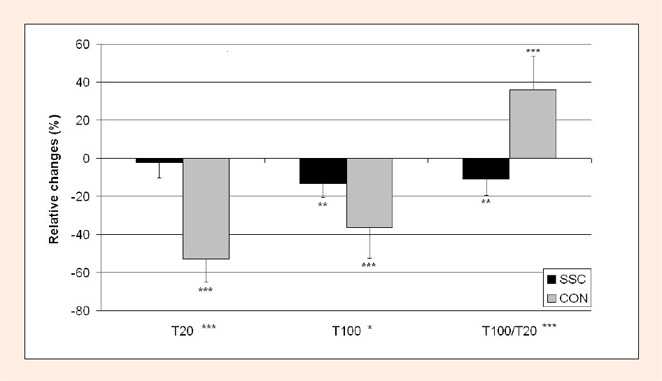Figure 1. T - torque at 100 Hz electrical stimulation; T - torque at 20 Hz electrical stimulation; T/T-torque ratio; SSC-stretch-shortening cycle exercise; CON-concentric exercise; bar asterisks denote significant differences compared to the value before each exercise (* p < 0.05; ** p < 0.01; *** p < 0.001); asterisks on the x-axis denote the significance of time and exercise main effects; all the data are given as means with their standard deviations.